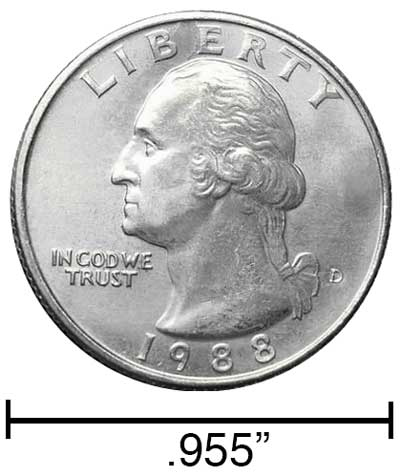# Meters to Inches Converter

Enter the length in meters below to get the value converted to inches.

Results in Inches:1 m = 39.370079 in
1 m ≈ 39 3/8"
*inch fraction is rounded to nearest 1/64"

Do you want to convert inches to meters?

## How to Convert Meters to Inches

To convert a measurement in meters to a measurement in inches, multiply the length by the following conversion ratio: 39.370079 inches/meter.

Since one meter is equal to 39.370079 inches, you can use this simple formula to convert:

inches = meters × 39.370079

The length in inches is equal to the length in meters multiplied by 39.370079.

For example, here's how to convert 5 meters to inches using the formula above.
inches = (5 m × 39.370079) = 196.850394"

### How Many Inches Are in a Meter?

There are 39.370079 inches in a meter, which is why we use this value in the formula above.

1 m = 39.370079"

Our inch fraction calculator can add meters and inches together, and it also automatically converts the results to US customary, imperial, and SI metric values.

## What is a Meter?

According to the most recent 2019 definition, the meter is defined as the distance traveled by light in vacuum during a time interval with a duration of 1/299,792,458 of a second. One meter is equal to 100 centimeters, 3.28084 feet, or 39.37 inches.

The meter, or metre, is the SI base unit for length in the metric system. Meters can be abbreviated as m; for example, 1 meter can be written as 1 m.

## What is an Inch?

An inch is a unit of length equal to 1/12 of a foot or 1/36 of a yard. Because the international yard is legally defined to be equal to exactly 0.9144 meters, one inch is equal to 2.54 centimeters.

The inch is a US customary and imperial unit of length. Inches can be abbreviated as in; for example, 1 inch can be written as 1 in.

Inches can also be denoted using the symbol, otherwise known as a double-prime. Often a double-quote (") is used instead of a double-prime for convenience. A double-prime is commonly used to express 1 in as 1″.The standard ruler is 12 inches long and is a common tool for measuring a length in inches. Another frequently used tool to perform measurements in inches is a tape measure, which commonly comes in lengths from 6' - 35'. Other types of devices used to make measurements in inches include scales, calipers, measuring wheels, micrometers, yardsticks, and even lasers.

We recommend using a ruler or tape measure for measuring length, which can be found at a local retailer or home center. Rulers are available in imperial, metric, or a combination of both values, so make sure you get the correct type for your needs.

Need a ruler? Try our free downloadable and printable rulers, which include both imperial and metric measurements.

## Meter to Inch Conversion Table

Meter measurements converted to inches in decimal and fraction form
Meters Inches (decimal) Inches (fraction)
1 m 39.37" 39 3/8"
2 m 78.74" 78 47/64"
3 m 118.11" 118 7/64"
4 m 157.48" 157 31/64"
5 m 196.85" 196 27/32"
6 m 236.22" 236 7/32"
7 m 275.59" 275 19/32"
8 m 314.96" 314 61/64"
9 m 354.33" 354 21/64"
10 m 393.7" 393 45/64"
11 m 433.07" 433 5/64"
12 m 472.44" 472 7/16"
13 m 511.81" 511 13/16"
14 m 551.18" 551 3/16"
15 m 590.55" 590 35/64"
16 m 629.92" 629 59/64"
17 m 669.29" 669 19/64"
18 m 708.66" 708 21/32"
19 m 748.03" 748 1/32"
20 m 787.4" 787 13/32"
21 m 826.77" 826 49/64"
22 m 866.14" 866 9/64"
23 m 905.51" 905 33/64"
24 m 944.88" 944 7/8"
25 m 984.25" 984 1/4"
26 m 1,024" 1,024"
27 m 1,063" 1,063"
28 m 1,102" 1,102"
29 m 1,142" 1,142"
30 m 1,181" 1,181"
31 m 1,220" 1,220"
32 m 1,260" 1,260"
33 m 1,299" 1,299"
34 m 1,339" 1,339"
35 m 1,378" 1,378"
36 m 1,417" 1,417"
37 m 1,457" 1,457"
38 m 1,496" 1,496"
39 m 1,535" 1,535"
40 m 1,575" 1,575"

## References

1. International Bureau of Weights and Measures, The International System of Units, 9th Edition, 2019, https://www.bipm.org/documents/20126/41483022/SI-Brochure-9-EN.pdf
2. National Institute of Standards and Technology, Checking the Net Contents of Packaged Goods, Handbook 133 - 2019 Edition, https://nvlpubs.nist.gov/nistpubs/hb/2019/NIST.HB.133-2019.pdf